Courses

# Test: Mental Ability, Analytical & Logical Reasoning - 1 (2019-2019)

## 20 Questions MCQ Test UPSC Topic Wise Previous Year Questions | Test: Mental Ability, Analytical & Logical Reasoning - 1 (2019-2019)

Description
This mock test of Test: Mental Ability, Analytical & Logical Reasoning - 1 (2019-2019) for UPSC helps you for every UPSC entrance exam. This contains 20 Multiple Choice Questions for UPSC Test: Mental Ability, Analytical & Logical Reasoning - 1 (2019-2019) (mcq) to study with solutions a complete question bank. The solved questions answers in this Test: Mental Ability, Analytical & Logical Reasoning - 1 (2019-2019) quiz give you a good mix of easy questions and tough questions. UPSC students definitely take this Test: Mental Ability, Analytical & Logical Reasoning - 1 (2019-2019) exercise for a better result in the exam. You can find other Test: Mental Ability, Analytical & Logical Reasoning - 1 (2019-2019) extra questions, long questions & short questions for UPSC on EduRev as well by searching above.
QUESTION: 1

### Seeta and Geeta go for a swim after a gap of every 2 days and every 3 days respectively. If on 1st January both of them went for a swim together, when will they go together next? 

Solution:

L.C.M of 3 and 4 = 12
together, they will meet on 1 + 12 = 13th jan.

QUESTION: 2

### In a school, 60% students play cricket. A student who does not play cricket, plays football. Every football player has got a two-wheeler. Which of the following conclusions cannot be drawn from the above data? 1. 60% of the students do not have two-wheelers. 2. No cricketer has a two-wheeler. 3. Cricket players do not play football. Select the correct answer using the code given below : 

Solution:

Every football player has got a two-wheeler does not mean that no cricketer has a two-wheeler.
So, we can not find the exact number of students do not have two-wheelers.
Hence, conclusion (1) and (2) Can not be drawn from the data.

QUESTION: 3

### P, Q and R are three towns. The distance between P and Q is 60 km, whereas the distance between P and R is 80 km. Q is in the West of P and R is in the South of P. What is the distance between Q and R? 

Solution:

Distance between city Q and city R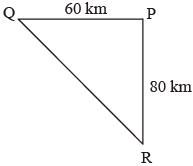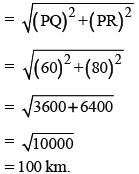QUESTION: 4

A five-storeyed building with floors from I to V is painted using four different colours and only one colour is used to paint a floor.
Consider the following statements :
1. The middle three floors are painted in different colours.
2. The second (II) and the fourth (IV) floors are painted in different colours.
3. The first (I) and the fifth (V) floors are painted red.
To ensure that any two consecutive floors have different colours



Solution:

From statement (3), floor 1 and 5 are painted by the same colour red.
Then, middle three floor 2, 3 and 4 must be painted in remaining three different colours.
Floor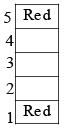In that way, we get that any two consecutive floor have different colours.

QUESTION: 5

Consider the following Statements and Conclusions:
Statements:
1. Some rats are cats.
2. Some cats are dog.
3. No dog is a cow.
Conclusions:
I. No cow is a cat.
II. No dog is a rat.
III. Some cats are rats.
Which of the above conclusions is/are drawn from the statements?



Solution: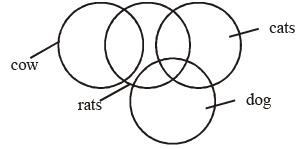Hence, some cats are rats is only conclusion that follows.

QUESTION: 6

Mr. ‘X’ has three children. The birthday of the first child falls on the 5th Monday of April, that of the second one falls on the 5th Thursday of November. On which day is the birthday of his third child, which falls on 20th December?

​

Solution:

5th monday of April will fall on 29th or 30th April. again 5th thursday of November will fall on 29th or 30th November.
When 29th April is Monday.
then, Number of days between 29th April and 29th November = 214 days
= 30 weeks + 4 days.
thus 29th of November would be Friday.
But 29th November is thursday, hence, 30th April is Monday.
Now, Number of days between 29th November and 20th december = 21 days.
= 3 weeks
Hence, 20th december is Thursday.

QUESTION: 7

Which year has the same calendar as that of 2009?



Solution:

Number of odd days in a non-Leap year = 1.
Number of odd days in a Leap year = 2.
Two year have same calender when number of odd days between two years is 7.
Between 2009 and 2015:
Number of non-leap year = 5 = 5 odd days
Number of Leap year = 1 = 2 odd days
Hence, 2015 have same calendar as 2009.

QUESTION: 8

Consider two statements S1 and S2 followed by a question:
S1 : p and q both are prime numbers.
S2 : p + q is an odd integer.
Question : Is pq an odd integer?
Which one of the following is correct?



Solution:

From statement S2, (p + q) is an odd integers.
i.e., p and q are not either even or odd simultaneously.
so, p and q are even and odd respectively and vice-versa.
Hence, p.q is always odd is incorrect. p.q is always even.

QUESTION: 9

‘A’ started from his house and walked 20 m towards East, where his friend ‘B’ joined him. They together walked 10 m in the same direction. Then ‘A’ turned left while ‘B’ turned right and travelled 2 m and 8 m respectively. Again ‘B’ turned left to travel 4 m followed by 5 m to his right to reach his office. ‘A’ turned right and travelled 12 m to reach his office. What is the shortest distance between the two offices?



Solution: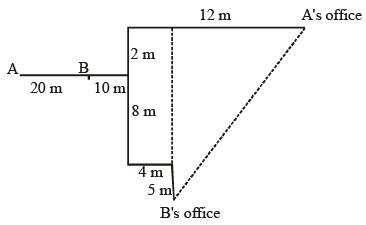Shortest distance between A and B.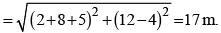QUESTION: 10

Consider the following sequence that follow some arrangement:
c_accaa_aa_bc_b
The letters that appear in the gaps are



Solution:

The series is ccacc, aabaa, bbcbb

QUESTION: 11

What is X in the sequence
4, 196, 16, 144, 36, 100, 64, X?



Solution: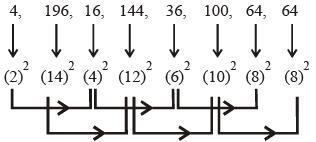This is double series sequence, here one series is increasing and other series is decreasing.

QUESTION: 12

If the numerator and denominator of a proper fraction are increased by the same positive quantity which is greater than zero, the resulting fraction is



Solution:

A proper fraction is one which is less than 1.
when some positive number is added in numerator and de-nominator then, fraction value increases from original value.
For example, when 1 is added to both numerator and denominator of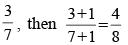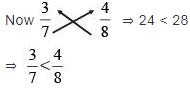QUESTION: 13

What is X in the sequence
132, 129, 124, 117, 106, 93, X?



Solution: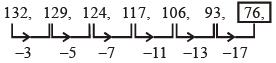QUESTION: 14

S1 : Twice the weight of Sohan is less than the weight of Mohan or that of Rohan.
S2 : Twice the weight of Rohan is greater than the weight of Mohan or that of Sohan.

Q. Which one of the following statements is correct?



Solution:

S1 → 2 × (Sohan's weight) < {(Mohan's weight) or (Rohan's weight)}
S2 → 2 × (Rohan's weight) > {(Mohan's weight) or (Sohan's weight)}
From S1, weight of Sohan is least.

QUESTION: 15

S1 : Twice the weight of Sohan is less than the weight of Mohan or that of Rohan.
S2 : Twice the weight of Rohan is greater than the weight of Mohan or that of Sohan.

Q. Which one of the following statements is correct?



Solution:

S1 → 2 × (Sohan's weight) < {(Mohan's weight) or (Rohan's weight)}
S2 → 2 × (Rohan's weight) > {(Mohan's weight) or (Sohan's weight)}
From S1 and S2, either Rohan or Mohan's weight is greatest but we can't determined whose weight is greatest.

QUESTION: 16

Six students A, B, C, D, E and F appeared in several tests. Either C or F scores the highest. Whenever C scores the highest, then E scores the least, Whenever F scores the highest, B scores the least.
In all the tests they got different marks; D scores higher than A, but they are close competitors; A scores higher than B; C scores higher than A.

Q. If F stands second in the ranking, then the position of B is



Solution:

Case - I: When C gets highest marks,
(i) E < F < B < A < D < C   or,
(ii) E < B < F < A < D < C   or,
(iii) E < B < A < D < F < C.
Case - II: When F Scores highest,
(i) B < A < D < C < E < F.  or,
(ii) B < A < D < E < C < F.
From case I (iii), When F stand second B is fifth.

QUESTION: 17

Six students A, B, C, D, E and F appeared in several tests. Either C or F scores the highest. Whenever C scores the highest, then E scores the least, Whenever F scores the highest, B scores the least.
In all the tests they got different marks; D scores higher than A, but they are close competitors; A scores higher than B; C scores higher than A.

Q. If B scores the least, the rank of C will be



Solution:

Case - I: When C gets highest marks,
(i) E < F < B < A < D < C   or,
(ii) E < B < F < A < D < C   or,
(iii) E < B < A < D < F < C.
Case - II: When F Scores highest,
(i) B < A < D < C < E < F.  or,
(ii) B < A < D < E < C < F.
From Case II, When B score the least then C stand either second or third.

QUESTION: 18

Six students A, B, C, D, E and F appeared in several tests. Either C or F scores the highest. Whenever C scores the highest, then E scores the least, Whenever F scores the highest, B scores the least.
In all the tests they got different marks; D scores higher than A, but they are close competitors; A scores higher than B; C scores higher than A.

Q. If E is ranked third, then which one of the following is correct?



Solution:

Case - I: When C gets highest marks,
(i) E < F < B < A < D < C   or,
(ii) E < B < F < A < D < C   or,
(iii) E < B < A < D < F < C.
Case - II: When F Scores highest,
(i) B < A < D < C < E < F.  or,
(ii) B < A < D < E < C < F.
From case - II (ii), When E ranked third, then C score more then E.

QUESTION: 19

If \$ means ‘divided by’; @ means ‘multiplied by’; # means ‘minus’; then the value of 10#5@1\$5 is



Solution:

10 # 5 @ 1 \$ 5
= 10 – 5 × 1 ÷ 5 = 10 – 5 × (1/5) = 10 – 1 = 9.

QUESTION: 20

A joint family consists of seven members A, B, C, D, E, F and G with three females. G is a widow and sister-in-law of D’s father F, B and D are siblings and A is daughter of B. C is cousin of B. Who is E?
(a) Wife of F
(b) Grandmother of A
(c) Aunt of C
Select the correct answer using the code given below:



Solution:

According to the question,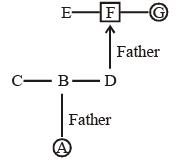So, E may be wife of F; Grandmother of A and Aunt of C
∴ Option (d) is correct.